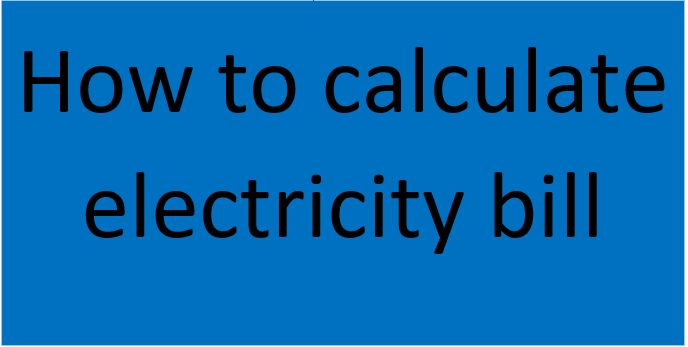# How to calculate electricity bill the best way in 2021I’m sharing the topic is how to calculate electricity bill and with this article, you can easily estimate your own monthly electricity bill.

For real information, firstly theoretical then practical side explanations will be done in this article. Firstly, I introduce electricity consumption it is a measurement of electricity and energy and it shows how much energy is used for calculating electric consumption at home.

## How to calculate electricity bills?

Do you know how to calculate electricity bills? If you do not know anything about the calculation procedure of the electricity, no worries, in this article, I will discuss in detail the calculation procedure of the electricity bill. Many people are confused about the unit of an energy meter, so, I will tell you what the unit is. Furthermore, you will also know how to calculate the units and the bill we pay in the USD/BDT/Rs. I will explain to you by considering some electrical equipment in a home by taking an example.

The first thing, I need to clarify you about the unit of a meter. Usually, we pay the bill as per unit. Actually, there is no difference between KWH and the unit. If you find that you consumed 100 Units for your house that means you have consumed 100KWH. Kwh means kilowatt-hours.

We use a simple formula to calculate the unit of electricity measurement. In most cases, we simply minus the previous reading to the current reading of an energy meter to determine the electricity unit of a home/house. With this formula, we can determine how much amount of money will pay on a single unit of consumption. But, this is a general formula. You can use the below method to estimate your monthly electricity bills.

## Determination of power consumption

How we will calculate the electricity or what will be the monthly unit as per your electricity meter. To calculate the electricity bill as units/Kwh, first, we need to determine the electricity consumption of every piece of equipment. Suppose you have three fans, one TV, one micro-oven, one refrigerator, and five bulbs. If you use the fan for 12 hours, TV for 10 hours, oven for 2 hours, refrigerator for 24 hours, and five bulbs for 8 hours, then we need to estimate the total uses of this equipment by considering each watt of this electrical equipment. The detailed calculations are shown in the below table.

Here, I have assumed the wattage of every piece of equipment. Nevertheless, you can find the actual wattage at the technical specification of the manufacturing manual or at the sticker, which is attached to the equipment. After calculating all the consumptions, it is found the total KWH/Unit is 8060/1000=8.06 Kwh/unit in a day. Since our monthly bills calculate considering 30 days, so, we need to multiply this unit by 30 days. Hence, we will get, 241.8Kwh or 241.8units. The usage time of the electrical equipment can be varied from time to time. For example, the fan load is used more time during the summer season. On the other hand, it is used less during the winter season. If you use 3 hours at any time, then your energy meter will be calculated according to 3 hours, sometimes if you use 8 hours, the energy meter will calculate the electricity bills considering for 8 hours.

## Difference between summer and winter season load for how to calculate electricity bill

I am also showing the difference in energy consumption between the summer as well as winter seasons. In the above table, I have considered the summer season whereas, in the winter season, we do not require any fan load due to the cold weather. Therefore, the total load consumption would be as follows for the winter season.

We can compare these two energy consumptions using a bar chart as shown below.

From the comparison chart, we can easily find that the summer energy consumption is slightly higher than the winter season. Therefore, during the summer season, your monthly bill will be greater than the normal winter season because at that time you consume more energy. Similarly, your monthly bill may vary from one month to another due to the fact that if you use some more electrical equipment in any month, then your energy consumption will be increased. On the other hand, if you use less electricity for your electrical equipment, then your monthly charge will be decreased.

## Conclusion

Different countries have different rates for each unit, even different cities in a country have different categories to pay the electricity charge. However, you need to search online about your local electricity supplier where you can get the unit charge. In addition to the electricity consumption/unit/kwh, some governments can charge additional tax. As an example, the electricity bill may equal energy charges plus fix charges plus meter rent plus demand charges plus surcharges. So, we should check all these things during calculating energy charges.

To find the surcharge or other things, you can check your electricity billing paper and you can also search online by the name of your local electricity billing authority. To know more, you can visit Wikipedia.

You can also read the below articles-

Analog to Digital Converter
Analog to digital converter – ADC, A/D, or A-to-D – is a device that converts analog signals into digital